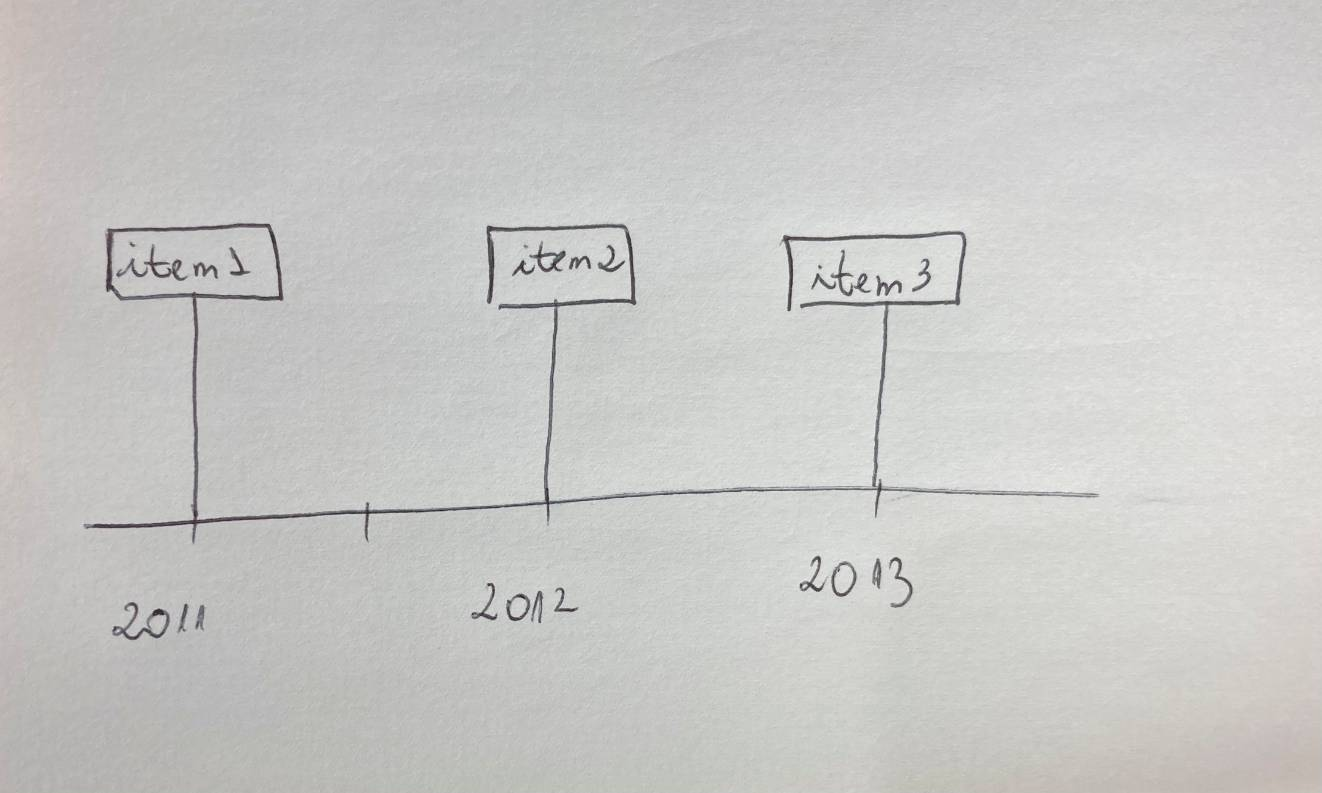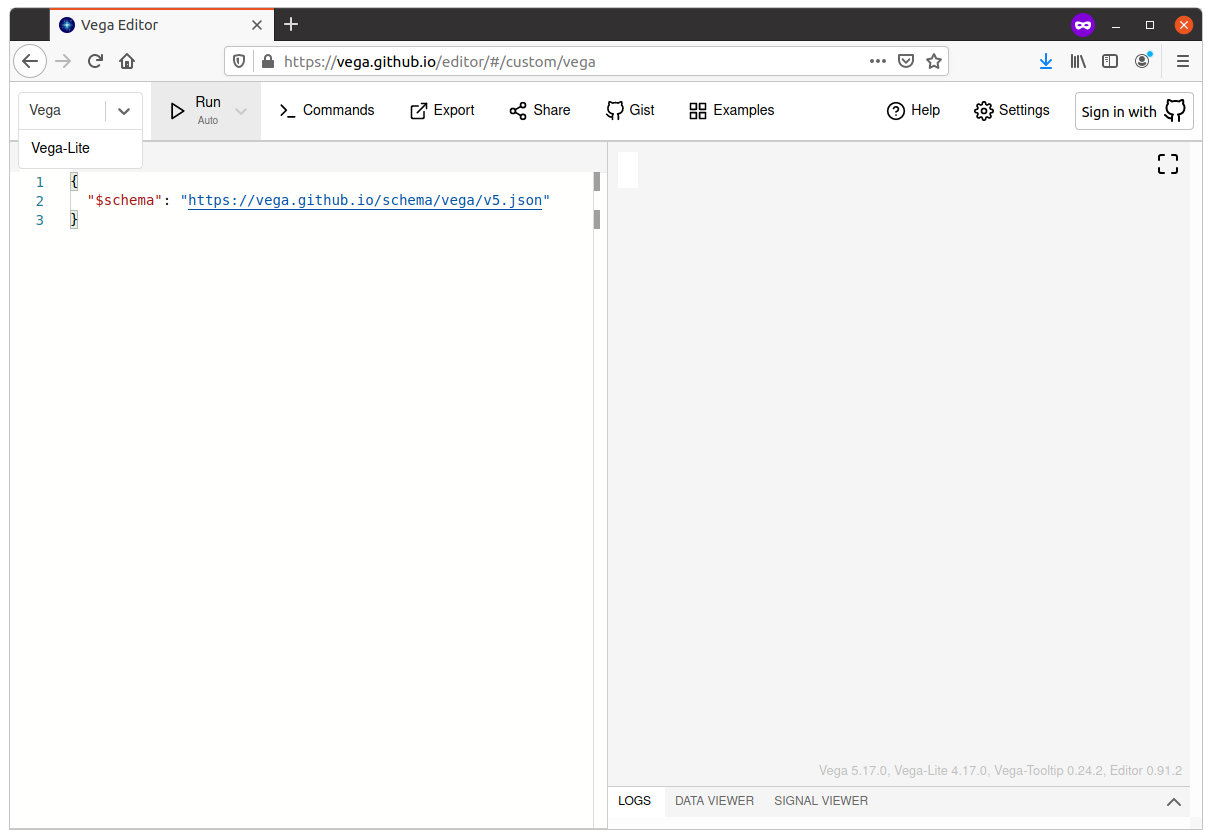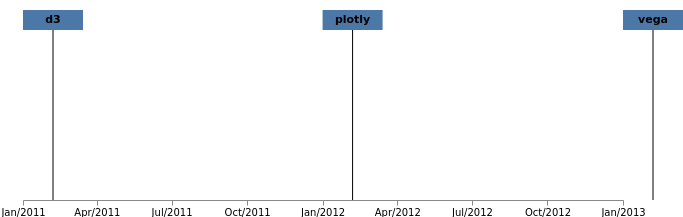## How to create a graph with Vega

Vega is a tool that introduces a visual grammar to build and manipulate visual elements. In this article you’ll learn how to go from an idea to an interactive graph with Vega. You specify the graph directives in a json file and in this tutorial you’ll learn how to build a timeline graph using the vega editor.

Enough said, let’s get started!

## Step 1 – draw the graph by hand

Yeah, you read it right. Just grab a piece of paper and draw the graph you want to build. Today you’ll build a timeline, like this:## Step 2 – break down the elements of your graph

Now you’ll list every element you see in your drawing. These are the elements of the above image:

• the rectangles for each item
• the text for each item
• the line from the rectangle to the x-axis
• the x-axis

## Step 3 – define how the main element of the graph will be set on the screen

The main elements of the timeline are the rectangles. They’ll be placed on the screen according to the year of the item.

## Step 4 – define your data and load it into Vega

This is where you’ll start coding. Since the rectangles will be placed according to the year, the data will look like this:

``````{"name":"vega", "release":"2013"},
{"name":"d3","release":"2011",
{"name":"plotly","release":"2012"}
``````

Now you will open the vega editor: https://vega.github.io/editor/#/. You put the code in the left panel. We’ll use Vega instead of Vega-Lite so don’t forget to change the specification there in the top-left corner.First and the size of the graph using the “witdh” and “height” parameters. Then add the data using the “data” parameter (notice the other parameters as well, such as the “type” of the data and how we parse the dates):

``````{
"\$schema": "https://vega.github.io/schema/vega/v5.json",
"width": 600,
"height": 200,
"data":[
{ "name": "visualization_libraries",
"format": {
"type": "json",
"parse": {
"release": "date:'%Y'"
}
},
"values": [
{"name":"vega", "release":"2013"},
{"name":"d3","release":"2011"},
{"name":"plotly","release":"2012"}
]
}
]
}
``````

## Step 5 – create a scale to place the main element of the graph

You’ll create a scale to place the rectangles. To do it you’ll use the “scales” parameter. You’ll name it “x_scale” and it will be a time scale. In “domain” you define where the data will come from, and in “range” you define the minimum and maximum values that will appear on the screen.

``````"scales": [
{
"name": "x_scale",
"type": "time",
"domain": {"data": "visualization_libraries","field": "release"},
"range": [0, 600],
"nice": "year"
}
]
``````

## Step 6 – place the main element of the graph on the screen

Time to place the rectangles. You’ll do it using the “marks” parameter, defining the type as “rect” and using x_scale to place them:

``````  "marks":[
{
"type": "rect",
"name": "rectangles",
"from": {"data": "visualization_libraries"},
"encode": {
"enter": {
"width": {"value": 60},
"height": {"value": 20},
"x": {
"signal": "scale('x_scale', datum.release)"
},
"y": {"value": 10}
}
}
}
]
``````

## Step 7 – add the other elements

You still need to add these elements:

• the rectangles for each item
• the text for each item
• the line from the rectangle to the x-axis
• the x-axis

First you’ll add the text. With Vega you can use the data from other marks as well, so this time you’ll use the data from the “rectangles” instead of “visualization_libraries” because this way you can simply get the x value from rectangle instead of computing the value from the scale again:

``````{
"type": "text",
"name": "labels",
"from": {"data": "rectangles"},
"encode": {
"enter": {
"text": {"signal": "datum.datum.name"},
"x": {
"signal": "datum.x+30"
},
"y": {"value": 20},
"align": {"value": "center"},
"baseline": {"value": "middle"},
"fontWeight": {"value": "bold"},
"fill": {"value": "black"}
}
}
}
``````

Now add the lines. This time using the data from “labels”:

``````{
"type": "rule",
"name": "lines",
"from": {"data": "labels"},
"encode": {
"enter": {
"x": {"signal": "datum.x"},
"x2": {"signal": "datum.x"},
"y": {"signal": "datum.y+10"},
"y2": {"signal": "height"}
}
}
}
``````

And finally place the axis using the “axes” parameter:

``````"axes":[
{
"scale": "xScale",
"orient": "bottom",
"format": "%b/%Y"
}
]
``````

And you’re done!You can check the whole code here in this gist.

In this quick tutorial you learned some Vega concepts but there is much more to learn. A good way to explore and dive deep is to check the examples: https://vega.github.io/vega/examples/